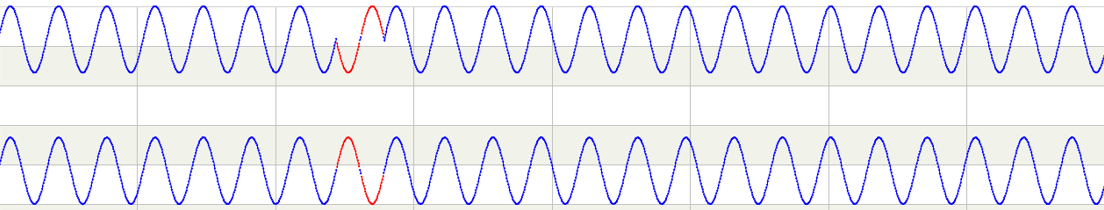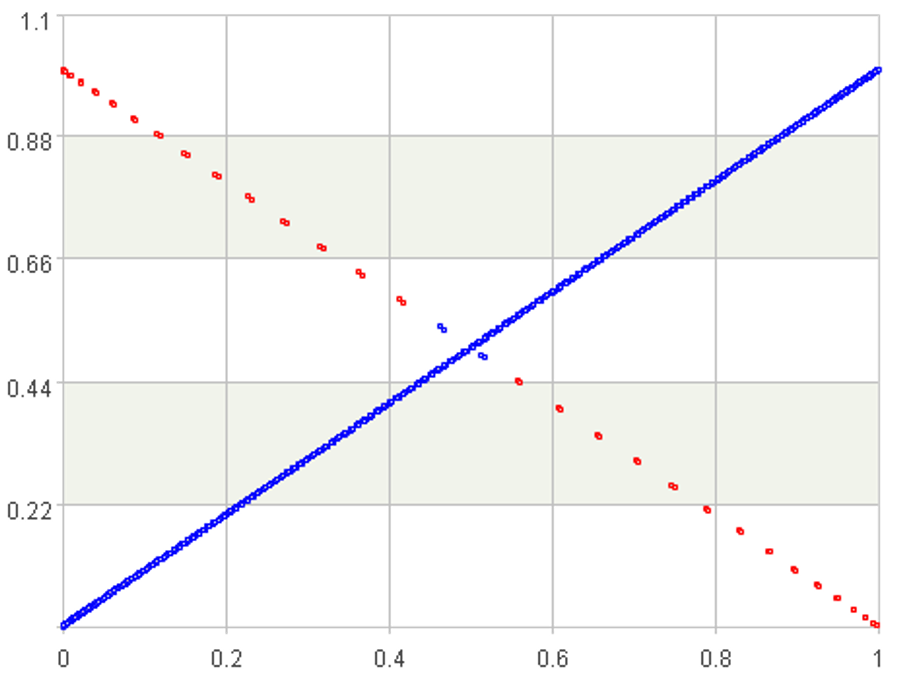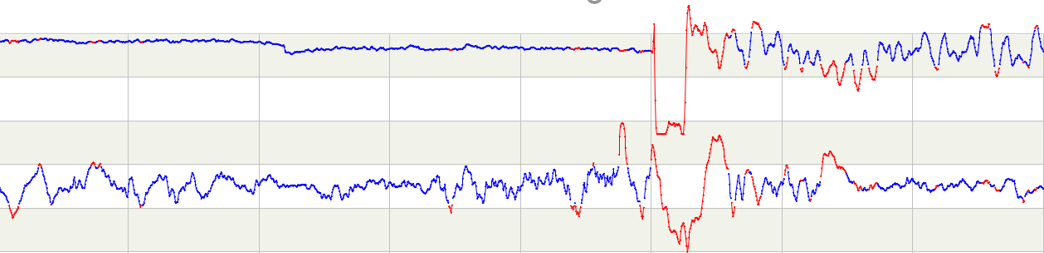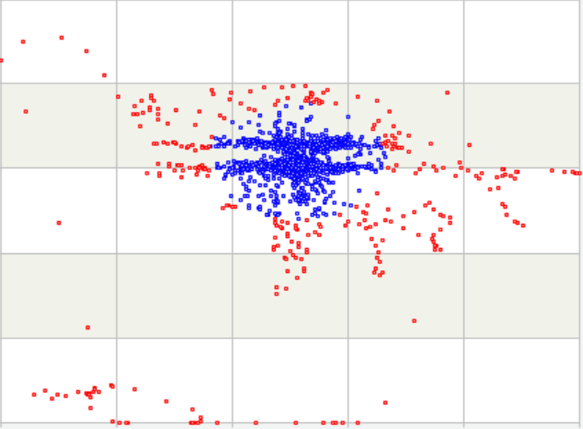## 基本问题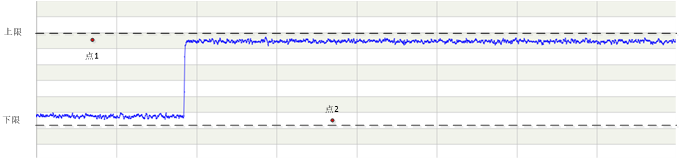### 算法思路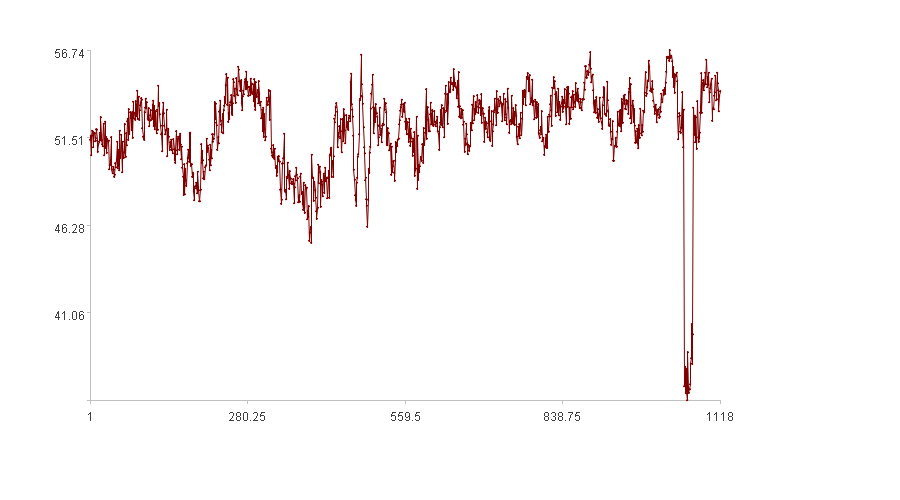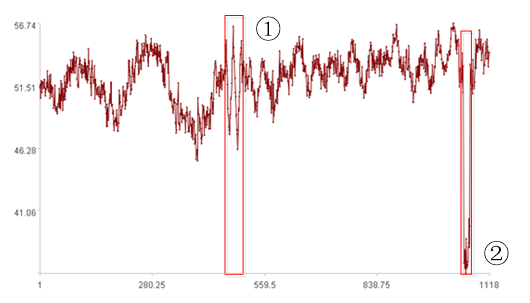① 变化过快；

② 值过小。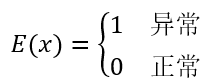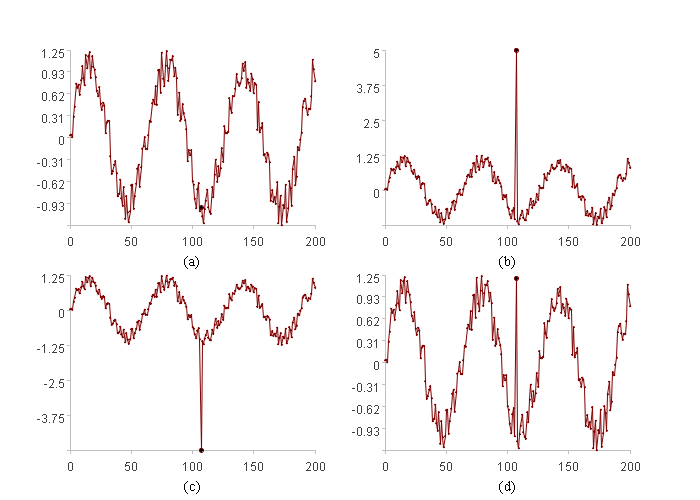(d)中的情况就是变化过快，我们来看看能否用“变化快慢”这个数学量来发现异常。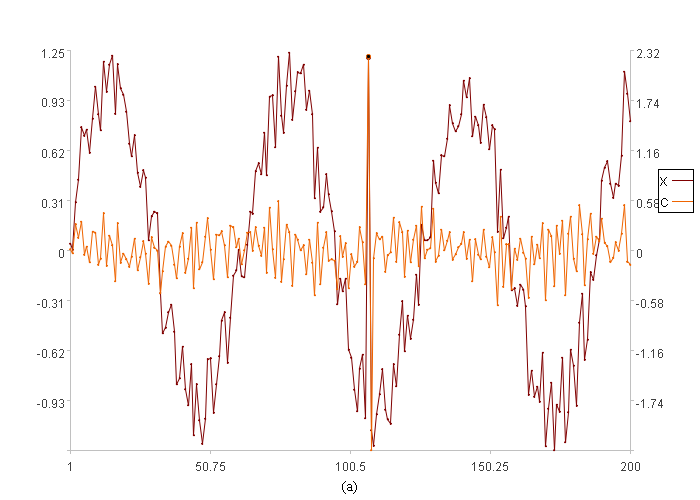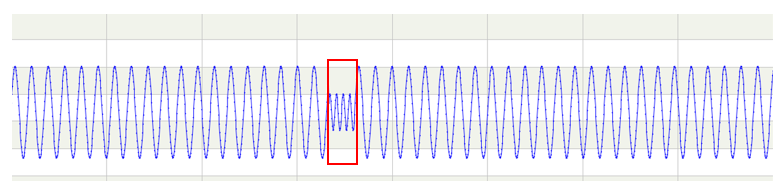### 实践效果

 A 1 =file(C1)[email protected]().(tag1) 2 =A1.(if(#<=100,,threshold(~[-100:0],"up",4))) 3 =A1.(if(#<=100,,threshold(~[-100:0],"down",4))) 4 =A1.m(101:) 5 =A2.m(101:) 6 =A3.m(101:) 7 =A4.(max(0,~-A5(#),A6(#)-~)/(A5(#)-A6(#)))

SPL有很强大的集合运算能力，实现区间上的阈值计算很方便。这段代码仅是个示意，并不完整，其中还调用了计算阈值的函数，而这个函数在不同场景要使用不同的计算方法，很难通用起来。这个问题足够大，值得专门撰文讨论，但并不是本文重点，所以就不再列出了，这并不影响理解其中的原理。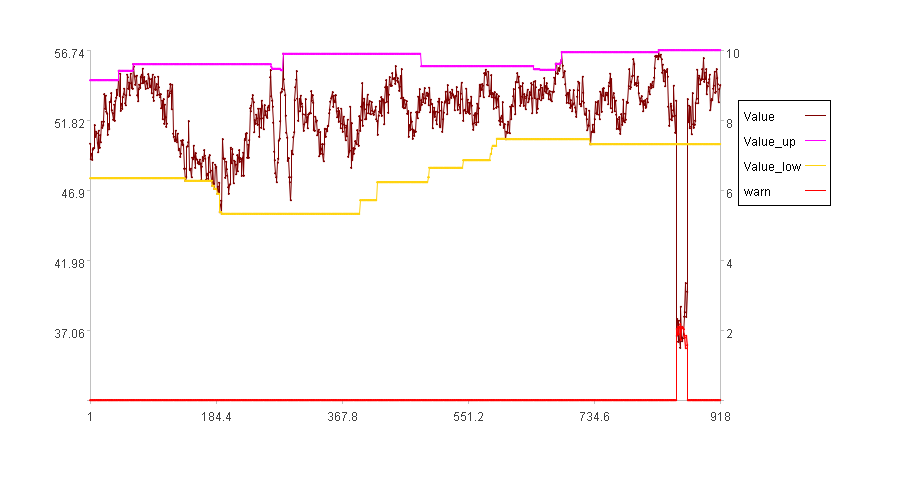A 1 =file(C1)[email protected]() 2 =A1.((ss=if(#<=D1,~[0:D1],~[-D1:0]),slope(ss))) 3 =A2.(if(#<=D2,,threshold(~[-D2:0],"up",3))) 4 =A2.(0) 5 =A2.m(D2+1:) 6 =A3.m(D2+1:) 7 =A4.m(D2+1:) 8 =A5.(max(0,~-A6(#),A7(#)-~)/(A6(#)-A7(#)))

“变化快慢”发现异常结果如下：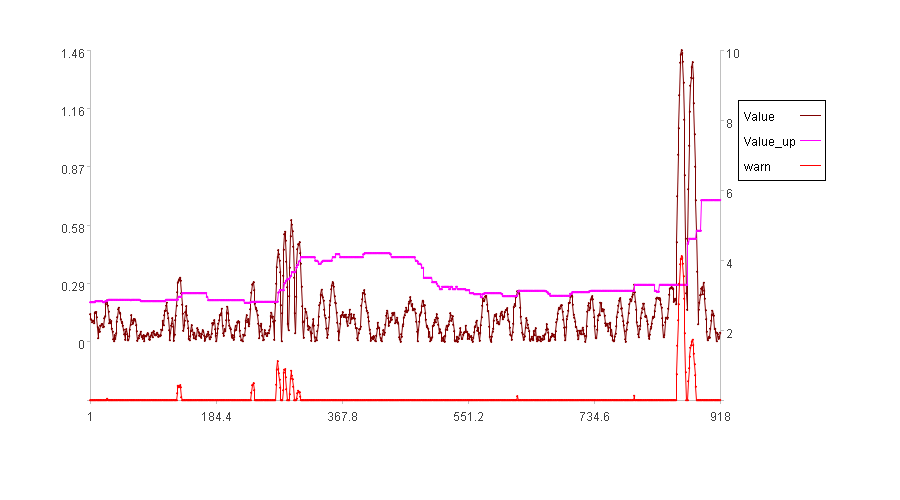## 关联问题

### 算法思路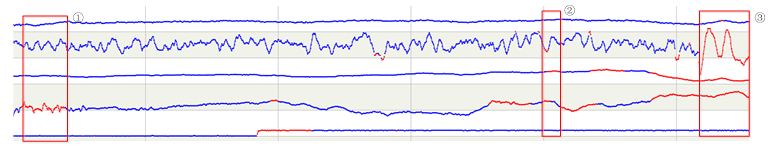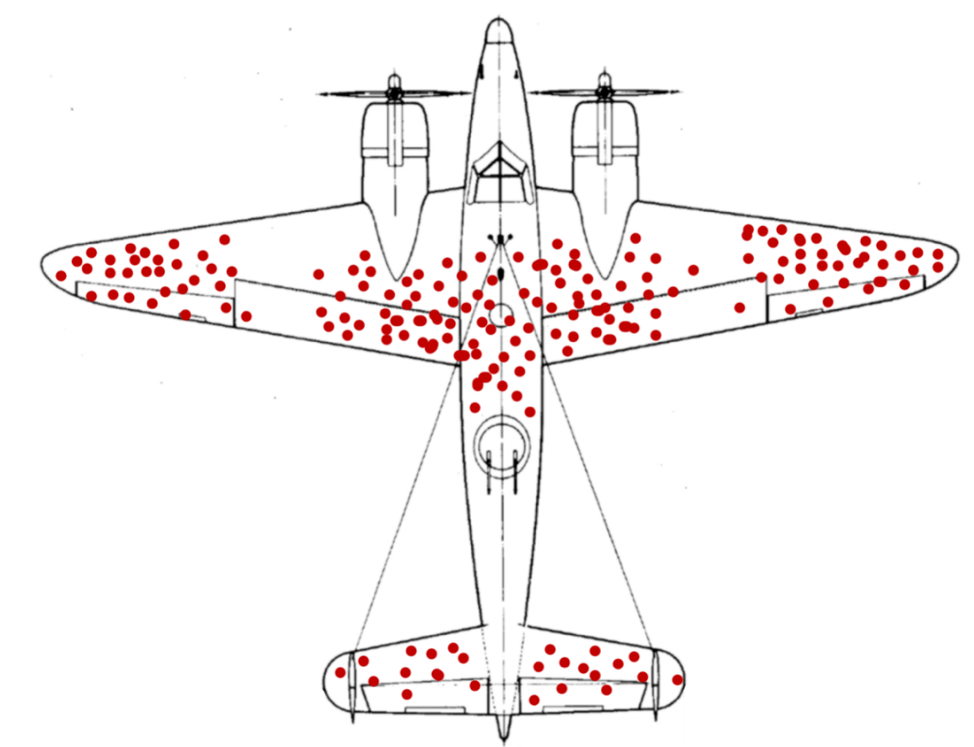### 实践效果

 A 1 =file("Adata.csv").read() 2 =A1.(~.array()) 3 =A2.(if(#<=100,null,weight(A14,~[-100:0]))) 4 =A2.to(101:) 5 =A3.to(101:) 6 =A4.((~**A5(#)).sum())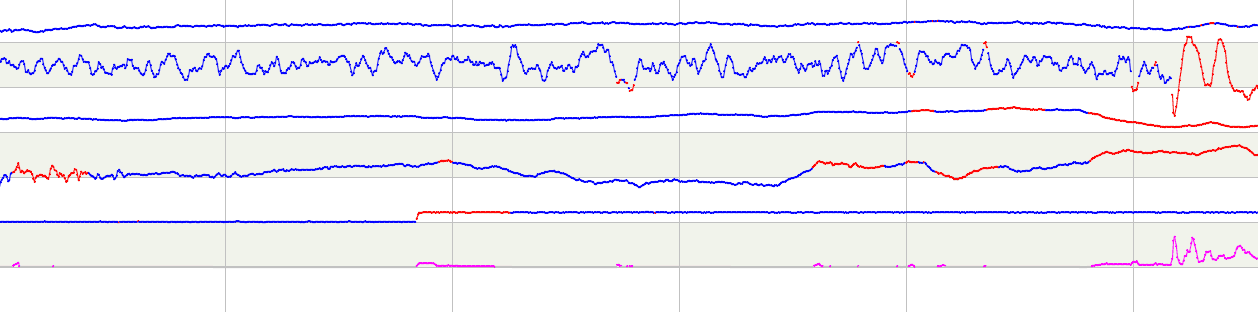## 进一步的关联问题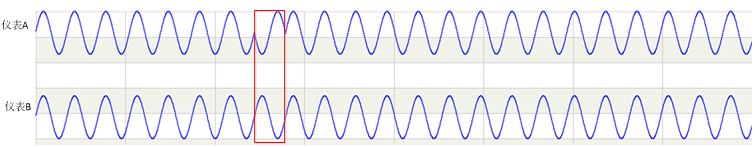### 算法思路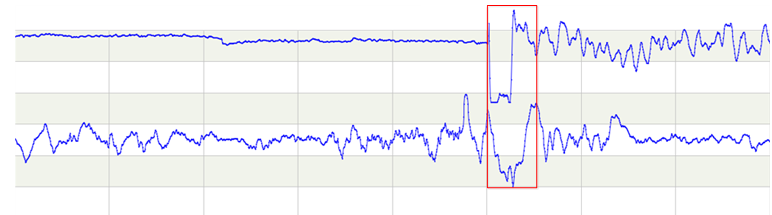### 实践效果

 A 1 =file("Jdata.csv").read() 2 =A1.(~.array()) 3 =A2.(if(#<=100,,join_o(~[-100:0],0.7,1.5))) 4 =A3.to(101,) 5 =A4.(if(~(1)>~(2),0,(~(2)-~(1))/~(2)))

A3格中关联方法同样要根据情况来确定。